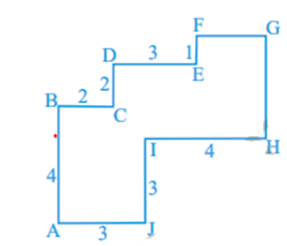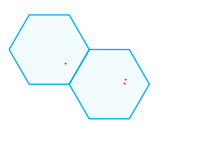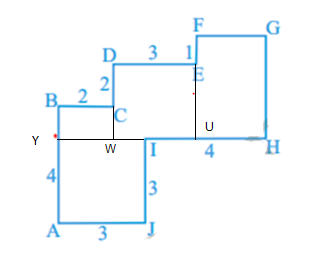# Important Questions for Class 6 Maths Mensuration

In this page we have Important Questions/worksheet for Class 6 Maths mensuration . Hope you like them and do not forget to like , social share and comment at the end of the page.
Question 1
Find the perimeter of each of the following shapes:
(a) A triangle of sides 12 cm, 5 cm and 13 cm.
(b) An equilateral triangle of side 8 cm.
(c) An isosceles triangle with equal sides 4 cm each and third side 3 cm.
(d) An square of side 10 cm
(e) An rectangle of side 11,10 cm
(f) triangle with sides measuring 10 cm, 14 cm and 15 cm.
(g) Regular hexagon of side 8 cm
(h) Regular pentagon of side 5 cm
Question 2
Solve the following
(a) Two sides of a triangle are 12 cm and 14 cm. The perimeter of the triangle is 36 cm. What is its third side?
(b) The perimeter of square is 40 cm, what is the side length?
(c) An equilateral triangle of perimeter 90 cm, what is the side length?
(d) The length of the rectangle is 10 cm and perimeter is 30 cm, what is the other side
Question 3
Find the perimeter of a rectangle whose length and breadth are 150 cm and 1 m respectively.
Question 4
Sachin takes 10 rounds of a rectangular park, 50 m long and 20 m wide. Find the total distance covered by him.
Question 5
Find the distance travelled by Naina if she takes three rounds of a square park of side 60 m.
Question 6
Find the perimeter of a regular pentagon with each side measuring 6 cm.
Question 7
The lid of a rectangular box of sides 40 cm by 10 m is to be sealed all round with tape. What is the length of the tape required?
Question 8
Find the areas of the rectangles whose sides are:
(a) 7 cm and 4 cm
(b) 2 m and 21 m
(c) 11 m and 1 m
(d) 2 m and 10 cm
Question 9
Find the areas of the squares whose sides are:
(a) 11 cm
(b) 25 cm
(c) 12 m
Question 10
Jatin wants to cover the floor of a room 3 m wide and 4 m long by squared tiles. If each square tile is of side 0.5 m, then find the number of tiles required to cover the floor of the room.
Question 11
Find the area in square meter of a piece of cloth 1m 25 cm wide and 2 m long.
Question 12
A room is 4 m long and 3 m 50 cm wide. How many square meters of carpet is needed to cover the floor of the room.
Question 13
The area of a rectangular garden 50 m long is 300 sq m. Find the width of the garden.
Question 14
By splitting the figure into rectangles, find its areaQuestion 15
Two regular Hexagons of perimeter 30cm each are joined as shown in below figure. Find the perimeter of the new figure(1)
These are straight forward question using the below formula of perimeter of rectangle,triangle,square
Perimeter of a rectangle = $2 \times (length + breadth)$
Perimeter of a square = $4 \times \text {length of its side}$
Perimeter of an equilateral triangle = $3 \times \text{length of a side}$
Perimeter of an triangle = $\text {sum of the lenght of the sides}$
(a) 30 cm
(b) 24 cm
(c) 11cm
(d) 40 cm
(e) 42 cm
(f) 39 cm
(g) 48 cm
(h) 25 cm
(2)
(a) 10 cm as third side= Perimeter - sum of the other two sides
(b) 10 cm as side of square = perimeter /4
(c) 30 cm as side of equilateral triangle = Perimeter/3
(d) 5 cm as Lenght + breadth = Perimter/2
(3) As Perimeter of a rectangle = $2 \times (length + breadth)$
Perimeter=302 m
(4) Distance= $10 \times Perimeter$
Now perimeter = 2(50+20) = 140m
Therefore,Distance= 1400m
(5) Distance= $3 \times Perimeter$
Now perimeter = 4*60 = 240m
Therefore,Distance= 720 m
(6) Pentagon has five equals sides So perimeter =$5 \times \text{lenght of the side} = 5 \times 6=30 cm$
(7) 100 m
(8) Area of rectangle is given by =$L \times B$
(a) 28 sq cm
(b) 42 sq m
(c) 11 sq m
(d) .2 sq m

(9) Area of square is given by =$side \times side$
(a) 121 sq cm
(b) 625 sq cm
(c) 144 sq m

(10) Area of the floor = Lenght × width= 12 sq m
Area of single tile=.5 × .5 = .25 sq m
Number of times required= 12 /.25 = 48

(11) Here we need to convert both the lenght and width into same unit
So ,lenght = 2 m
Width=1m 25 cm=1.25 m
Area= 2 × 1.25 = 2.5 sq m

(12) L=4 m
W=3 m 50 cm =3.5 m
Area of carpet = 4 * 3.5 = 14 sq m

(13) Area= 300 sq m
length =50 m
Area = Lenght × width= 50 × width
Therefore,
50 × width =300
width= 6 m

(14) Splitting the figure as belowArea of the figure = Area AJIY + Area YWCB + Area DWUE + Area FUHG.
Area A J I Y = A J * J I = 3 * 3 = 9
Now, B Y = A B - Y A = 4 - 3 = 1
So, Area Y W C B = B Y * B C = 1 * 2 = 2
Next, D W = D C + C W = 2 + 1 = 3
Therefore, area D W U E = D W * D E = 3 * 3 = 9
Similarly, U H = I H - I U = 4 - 2 = 2
G H = F U and F U = E U + F E
= D W + F E = 3 + 1= 4
Area F U H G = U H * G H = 2 * 4 = 8
Therefore, the area of the figure = 9 + 2 + 9 + 8
= 28sq units

(15) Side of Hexagon = perimeter / 6 = 30/6 =5 cm
Now Perimeter of the given figure= 10 × side = 50 cm### Practice Question

Question 1 What is $\frac {1}{2} + \frac {3}{4}$ ?
A)$\frac {5}{4}$
B)$\frac {1}{4}$
C)$1$
D)$\frac {4}{5}$
Question 2 Pinhole camera produces an ?
A)An erect and small image
B)an Inverted and small image
C)An inverted and enlarged image
D)None of the above